### 4.4.3 Well-Formedness Clauses

Procedure `Clauses` creates the well-formedness clauses for the pair of variablesand, where `N1` denotes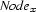, `N2` denotes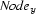, and `R` denotes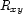. Note that we identifywith 1,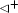with 2,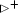with 3, and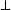with 4.

`proc {Clauses N1 N2 C}   thread or {Equal    N1 N2} C=1 [] C\=:1 {NotEqual    N1 N2} end end    thread or {Above    N1 N2} C=2 [] C\=:2 {NotAbove    N1 N2} end end    thread or {Above    N2 N1} C=3 [] C\=:3 {NotAbove    N2 N1} end end    thread or {Disjoint N1 N2} C=4 [] C\=:4 {NotDisjoint N1 N2} end end end`

Denys Duchier
Version 1.2.0 (20010221)